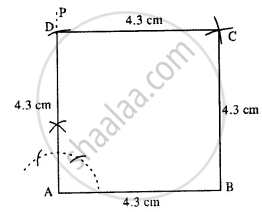# Construct a Square, If : Its Each Side is 4.3 Cm. - Mathematics

Sum

Construct a square, if :

its each side is 4.3 cm.

#### SolutionSteps :

1. Draw AB = 4.3 cm.
2. Draw ∠PAB = 90° at A.
3. Cut AD = 4.3 cm.
4. From B and D, draw arcs of radii 4.3 cm each which intersect at C.
5. Join AD, BC, and CD.
Hence ABCD is the required square.
Concept: Construction of Square
Is there an error in this question or solution?

#### APPEARS IN

Selina Concise Mathematics Class 8 ICSE
Chapter 18 Constructions
Exercise 18 (D) | Q 5.2 | Page 211